# 图像处理 用python

## 教你用Python做图像处理

2018-11-18 15:02:40 qq_37482202 阅读数 19895
• ###### Python+OpenCV3.3图像处理视频教程

Python+OpenCV3.3图像处理视频培训课程：该教程基于Python3.6+OpenCV新版本3.3.0详细讲述Python OpenCV图像处理部分内容，包括opencv人脸识别、人脸检测、数字验证码识别等内容。是Python开发者学习图像知识与应用...

30课时 663分钟 6356人学习 贾志刚
免费试看

# 1.open()

image = Image.open(image_address)

# 2.covert()

im.convert(mode) ⇒ image

im.convert(“P”, **options) ⇒ image

im.convert(mode, matrix) ⇒ image

## 2.模式“L”

L = R * 299/1000 + G * 587/1000+ B * 114/1000

## 6.模式“YCbCr”

Y= 0.257*R+0.504*G+0.098*B+16
Cb = -0.148*R-0.291*G+0.439*B+128
Cr = 0.439*R-0.368*G-0.071*B+128

## 7.模式“I”

I = R * 299/1000 + G * 587/1000 + B * 114/1000

## 8.模式“F”

F = R * 299/1000+ G * 587/1000 + B * 114/1000

# 3.调整尺寸、创建缩略图、裁剪、贴图、旋转

PIL库给我们提供了丰富基本图像操作，如果你想调整一张图片的尺寸，你可以使用resize()方法，该方法需要传入你指定新图像宽高的元组

img = img.resize((128,128))

img=img.thumbnail((128,128))

box = (100,100,400,400)
img = img.crop(box)

img2=img2.paste(img1,(100,100,200,200))

img = img.transpose(Image.ROTATE_180)

• PIL.Image.FLIP_LEFT_RIGHT
• PIL.Image.FLIP_TOP_BOTTOM
• PIL.Image.ROTATE_90
• PIL.Image.ROTATE_180
• PIL.Image.ROTATE_270
• PIL.Image.TRANSPOSE
• PIL.Image.TRANSVERSE

image = image.rotate(45)

# 4.Numpy

image = Image.open(image_address)
imageArray = array(image)

## 1.反向处理与二值化

imageArray = 255 - imageArray

图像的二值化也很简单，0-255以128为分界，小于128置为0否则置为1

imageArray = 1 * (imageArray < 128)

## 2.像素值限制范围

imageArray = (100.0 / 255) * imageArray + 100

## 3.像素值求平方

imageArray = 255.0 * (imageArray / 255.0) ** 2

## 4.直方图均衡化

def histeq(im,nbr_bins=256):
""" 对一幅灰度图像进行直方图均衡化"""
# 计算图像的直方图
imhist,bins = histogram(im.flatten(),nbr_bins,normed=True)
cdf = imhist.cumsum()
# cumulative distribution function
cdf = 255 * cdf / cdf[-1]
#  归一化
#  使用累积分布函数的线性插值，计算新的像素值
im2 = interp(im.flatten(),bins[:-1],cdf)
return im2.reshape(im.shape), cdf

## 5.多种滤波

gaussian滤波是多维的滤波器，是一种平滑滤波，可以消除高斯噪声

imageArray = filters.gaussian_filter(imageArray, 5)

sobel算子可用来检测边缘

edges = filters.sobel(img)

roberts算子、scharr算子、prewitt算子和sobel算子一样，用于检测边缘

edges = filters.roberts(img)
edges = filters.scharr(img)
edges = filters.prewitt(img)

canny算子也是用于提取边缘特征，但它不是放在filters模块，而是放在feature模块

edges1 = feature.canny(img)   #sigma=1
edges2 = feature.canny(img,sigma=3)   #sigma=3

gabor滤波可用来进行边缘检测和纹理特征提取。

filt_real, filt_imag = filters.gabor_filter(img,frequency=0.6)   

## 6.PCA

PCA（Principal Component Analysis，主成分分析）是一个非常有用的降维技巧。它可以在使用尽可能少维数的前提下，尽量多地保持训练数据的信息，在此意义上是一个最佳技巧。即使是一幅 100×100 像素的小灰度图像，也有 10 000 维，可以看成 10 000 维空间中的一个点。一兆像素的图像具有百万维。由于图像具有很高的维数，在许多计算机视觉应用中，我们经常使用降维操作。PCA 产生的投影矩阵可以被视为将原始坐标变换到现有的坐标系，坐标系中的各个坐标按照重要性递减排列。

def pca(X):
""" 主成分分析：    输入：矩阵X ，其中该矩阵中存储训练数据，每一行为一条训练数据
返回：投影矩阵（按照维度的重要性排序）、方差和均值"""
# 获取维数
num_data,dim = X.shape
# 数据中心化
mean_X = X.mean(axis=0)
X = X - mean_X
if dim<num_data:
# PCA- 使用紧致技巧
M = dot(X,X.T)
# 协方差矩阵
e,EV = linalg.eigh(M)
# 特征值和特征向量
tmp = dot(X.T,EV).T
# 这就是紧致技巧
V = tmp[::-1]
# 由于最后的特征向量是我们所需要的，所以需要将其逆转
S = sqrt(e)[::-1]
# 由于特征值是按照递增顺序排列的，所以需要将其逆转
for i in range(V.shape):
V[:,i] /= S
else:
# PCA- 使用SVD 方法
U,S,V = linalg.svd(X)
V = V[:num_data]
# 仅仅返回前nun_data 维的数据才合理
#  返回投影矩阵、方差和均值
return V,S,mean_X

## 7.图像添加噪声和降噪

imageArray = imageArray + 30 * random.standard_normal(imageArray.shape)

def denoise(im,U_init,tolerance=0.1,tau=0.125,tv_weight=100):
""" 使用A. Chambolle（2005）在公式（11）中的计算步骤实现Rudin-Osher-Fatemi（ROF）去噪模型
输入：含有噪声的输入图像（灰度图像）、U 的初始值、TV 正则项权值、步长、停业条件
输出：去噪和去除纹理后的图像、纹理残留"""
m,n = im.shape # 噪声图像的大小

#  初始化
U = U_init
Px = im # 对偶域的x 分量
Py = im # 对偶域的y 分量
error = 1
while (error > tolerance):
Uold = U

# 原始变量的梯度
GradUx = roll(U,-1,axis=1)-U # 变量U 梯度的x 分量
GradUy = roll(U,-1,axis=0)-U # 变量U 梯度的y 分量

#  更新对偶变量
NormNew = maximum(1,sqrt(PxNew**2+PyNew**2))
Px = PxNew/NormNew # 更新x 分量（对偶）
Py = PyNew/NormNew # 更新y 分量（对偶）
#  更新原始变量
RxPx = roll(Px,1,axis=1) # 对x 分量进行向右x 轴平移
RyPy = roll(Py,1,axis=0) # 对y 分量进行向右y 轴平移

DivP = (Px-RxPx)+(Py-RyPy) # 对偶域的散度
U = im + tv_weight*DivP # 更新原始变量

#  更新误差
error = linalg.norm(U-Uold)/sqrt(n*m);
return U,im-U  # 去噪后的图像和纹理残余


# 5.Matplotlib

from PIL import Image
from pylab import *

# 读取图像到数组中
im = array(Image.open('empire.jpg'))

# 绘制图像
imshow(im)

# 一些点
x = [100,100,400,400]
y = [200,500,200,500]

# 使用红色星状标记绘制点
plot(x,y,'r*')

# 绘制连接前两个点的线
plot(x[:2],y[:2])

# 添加标题，显示绘制的图像
title('Plotting: "empire.jpg"')
show()

axis('off')

下面是我写的一个图像处理的脚本

import PIL.Image as Image
import os
from pylab import *
from numpy import *
from scipy.ndimage import filters
from scipy.ndimage import measurements,morphology

def get_imlist(path):
# 一级文件夹下有用
# return [os.path.join(path, f) for f in os.listdir(path) if f.endswith('.jpg')]
g = os.walk(path)
image_list=[]
for path, d, filelist in g:
for filename in filelist:
if filename.endswith('jpg'):
image_list.append(os.path.join(path, filename))
return image_list

def histeq(im,nbr_bins=256):
""" 对一幅灰度图像进行直方图均衡化"""
# 计算图像的直方图
imhist,bins = histogram(im.flatten(),nbr_bins,normed=True)
cdf = imhist.cumsum()
# cumulative distribution function
cdf = 255 * cdf / cdf[-1]
#  归一化
#  使用累积分布函数的线性插值，计算新的像素值
im2 = interp(im.flatten(),bins[:-1],cdf)
return im2.reshape(im.shape), cdf

def pca(X):
""" 主成分分析：    输入：矩阵X ，其中该矩阵中存储训练数据，每一行为一条训练数据
返回：投影矩阵（按照维度的重要性排序）、方差和均值"""
# 获取维数
num_data,dim = X.shape
# 数据中心化
mean_X = X.mean(axis=0)
X = X - mean_X
if dim<num_data:
# PCA- 使用紧致技巧
M = dot(X,X.T)
# 协方差矩阵
e,EV = linalg.eigh(M)
# 特征值和特征向量
tmp = dot(X.T,EV).T
# 这就是紧致技巧
V = tmp[::-1]
# 由于最后的特征向量是我们所需要的，所以需要将其逆转
S = sqrt(e)[::-1]
# 由于特征值是按照递增顺序排列的，所以需要将其逆转
for i in range(V.shape):
V[:,i] /= S
else:
# PCA- 使用SVD 方法
U,S,V = linalg.svd(X)
V = V[:num_data]
# 仅仅返回前nun_data 维的数据才合理
#  返回投影矩阵、方差和均值
return V,S,mean_X

def denoise(im,U_init,tolerance=0.1,tau=0.125,tv_weight=100):
""" 使用A. Chambolle（2005）在公式（11）中的计算步骤实现Rudin-Osher-Fatemi（ROF）去噪模型
输入：含有噪声的输入图像（灰度图像）、U 的初始值、TV 正则项权值、步长、停业条件
输出：去噪和去除纹理后的图像、纹理残留"""
m,n = im.shape # 噪声图像的大小

#  初始化
U = U_init
Px = im # 对偶域的x 分量
Py = im # 对偶域的y 分量
error = 1
while (error > tolerance):
Uold = U

# 原始变量的梯度
GradUx = roll(U,-1,axis=1)-U # 变量U 梯度的x 分量
GradUy = roll(U,-1,axis=0)-U # 变量U 梯度的y 分量

#  更新对偶变量
NormNew = maximum(1,sqrt(PxNew**2+PyNew**2))
Px = PxNew/NormNew # 更新x 分量（对偶）
Py = PyNew/NormNew # 更新y 分量（对偶）
#  更新原始变量
RxPx = roll(Px,1,axis=1) # 对x 分量进行向右x 轴平移
RyPy = roll(Py,1,axis=0) # 对y 分量进行向右y 轴平移

DivP = (Px-RxPx)+(Py-RyPy) # 对偶域的散度
U = im + tv_weight*DivP # 更新原始变量

#  更新误差
error = linalg.norm(U-Uold)/sqrt(n*m);
return U,im-U  # 去噪后的图像和纹理残余

image_list = get_imlist("G:\\最后两种\\")

index=6858
index = index + 1
dealIndex=0
for x in range(1,17):
imageArray = array(image)
dealIndex+=1
if x==1:
# 反相处理
imageArray = 255 - imageArray
print("第"+str(index)+"张 反向处理")
elif x==2:
# 将图像像素值变换到100...200 区间
imageArray = (100.0 / 255) * imageArray + 100
print("第" + str(index) + "张 像素值变换")
elif x==3:
# 对图像像素值求平方后得到的图像
imageArray = 255.0 * (imageArray / 255.0) ** 2
print("第" + str(index) + "张 像素值求平方")
elif x==4:
# 图像旋转
image = image.rotate(random.randint(0,360))
imageArray=array(image)
print("第" + str(index) + "张 图像旋转")
elif x==5:
# 直方图均衡化
imageArray,cdf=histeq(imageArray)
print("第" + str(index) + "张 直方图均衡化")
elif x==6:
# gaussian滤波
imageArray = filters.gaussian_filter(imageArray, 5)
print("第" + str(index) + "张 gaussian滤波")
elif x==7:
# Sobel 导数滤波器
imx = zeros(imageArray.shape)
filters.sobel(imageArray, 1, imx)
imy = zeros(imageArray.shape)
filters.sobel(imageArray, 0, imy)
magnitude = sqrt(imx ** 2 + imy ** 2)
imageArray=magnitude
print("第" + str(index) + "张  Sobel导数滤波器")
elif x==8:
# 噪声
imageArray = imageArray + 30 * random.standard_normal(imageArray.shape)
print("第" + str(index) + "张  噪声")
elif x==9:
# 反相处理+像素值变换
imageArray = 255 - imageArray
imageArray = (100.0 / 255) * imageArray + 100
print("第" + str(index) + "张  反相处理+像素值变换")
elif x==10:
# 反相处理+像素值求平方
imageArray = 255 - imageArray
imageArray = 255.0 * (imageArray / 255.0) ** 2
print("第" + str(index) + "张  反相处理+像素值求平方")
elif x==11:
# 像素值求平方+反相处理
imageArray = 255.0 * (imageArray / 255.0) ** 2
imageArray = 255 - imageArray
print("第" + str(index) + "张  像素值求平方+反相处理")
elif x==12:
# 像素值变换+像素值求平方
imageArray = (100.0 / 255) * imageArray + 100
imageArray = 255.0 * (imageArray / 255.0) ** 2
print("第" + str(index) + "张  像素值变换+像素值求平方")
elif x==13:
# 图像旋转+反相
image = image.rotate(random.randint(0, 360))
imageArray = array(image)
imageArray = 255 - imageArray
print("第" + str(index) + "张  图像旋转+反相")
elif x==14:
# 图像旋转+噪声
image = image.rotate(random.randint(0, 360))
imageArray = array(image)
imageArray = imageArray + 30 * random.standard_normal(imageArray.shape)
print("第" + str(index) + "张  图像旋转+噪声")
elif x==15:
# 噪声+直方图均衡化
imageArray = imageArray + 30 * random.standard_normal(imageArray.shape)
imageArray, cdf = histeq(imageArray)
print("第" + str(index) + "张  噪声+直方图均衡化")

imageArray = uint8(imageArray)
image=Image.fromarray(imageArray)
image = image.convert('RGB')
image.save("G:\\兔屎图片_二次处理\\不规则\\" + str(index)+"_"+str(dealIndex) + ".jpg")
image.save("G:\\兔屎图片_二次处理\\大小不一\\" + str(index) +"_"+str(dealIndex)+ ".jpg")
image.save("G:\\兔屎图片_二次处理\\拉稀\\" + str(index) +"_"+str(dealIndex)+ ".jpg")
image.save("G:\\兔屎图片_二次处理\\正常\\" + str(index) +"_"+str(dealIndex)+ ".jpg")

print("完事一个")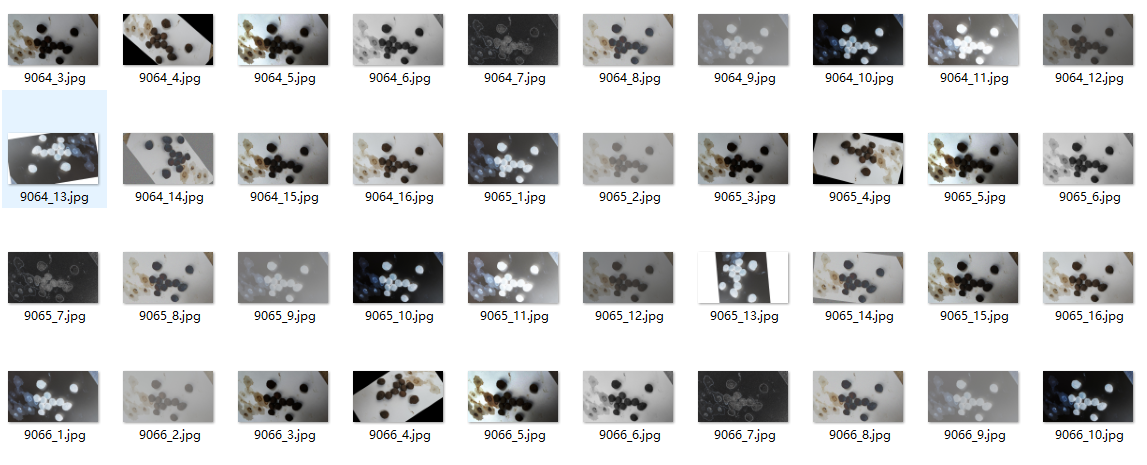## 用Python做图像处理

• ###### Python+OpenCV3.3图像处理视频教程

Python+OpenCV3.3图像处理视频培训课程：该教程基于Python3.6+OpenCV新版本3.3.0详细讲述Python OpenCV图像处理部分内容，包括opencv人脸识别、人脸检测、数字验证码识别等内容。是Python开发者学习图像知识与应用...

30课时 663分钟 6356人学习 贾志刚
免费试看

最近在做一件比较 evil 的事情——验证码识别，以此来学习一些新的技能。因为我是初学，对图像处理方面就不太了解了，欲要利吾事，必先利吾器，既然只是做一下实验，那用 Python 来作原型开发再好不过了。在 Python 中，比较常用的图像处理库是 PIL(Python Image Library)，当前版本是 1.1.6 ，用起来非常方便。大家可以在 http://www.pythonware.com/products/pil/index.htm 下载和学习。
在这里，我主要是介绍一下做图像识别时可能会用到的一些 PIL 提供的功能，比如图像增强、还有滤波之类的。最后给出使用 Python 做图像处理与识别的优势与劣势。

使用 PIL 之前需要 import Image 模块：
import Image
然后你就可以使用Image.open(‘xx.bmp’) 来打开一个位图文件进行处理了。打开文件你不用担心格式，也不用了解格式，无论什么格式，都只要把文件名丢给 Image.open 就可以了。真所谓 bmp、jpg、png、gif……，一个都不能少。
img = Image.open(‘origin.png’)    # 得到一个图像的实例对象 img1原图
图像处理中，最基本的就是色彩空间的转换。一般而言，我们的图像都是 RGB 色彩空间的，但在图像识别当中，我们可能需要转换图像到灰度图、二值图等不同的色彩空间。　PIL 在这方面也提供了极完备的支持，我们可以：
new_img = img.convert(‘L’)

· 1 (1-bit pixels, black and white, stored with one pixel per byte)
· L (8-bit pixels, black and white)
· P (8-bit pixels, mapped to any other mode using a colour palette)
· RGB (3x8-bit pixels, true colour)
· RGBA (4x8-bit pixels, true colour with transparency mask)
· CMYK (4x8-bit pixels, colour separation)
· YCbCr (3x8-bit pixels, colour video format)
· I (32-bit signed integer pixels)
· F (32-bit floating point pixels)2 mode = '1'3 mode = 'L'4 mode = 'P'
convert() 函数也接受另一个隐含参数 matrix，转换矩阵 matrix 是一个长度为4 或者16 tuple。下例是一个转换 RGB 空间到 CIE XYZ 空间的例子：
rgb2xyz = (
0.412453, 0.357580, 0.180423, 0,
0.212671, 0.715160, 0.072169, 0,
0.019334, 0.119193, 0.950227, 0 )
out = im.convert("RGB", rgb2xyz)
除了完备的色彩空间转换能力外， PIL 还提供了resize()、rotate()等函数以获得改变大小，旋转图片等几何变换能力，在图像识别方面，图像实例提供了一个 histogram() 方法来计算直方图，非常方便实用。

图像增强通常用以图像识别之前的预处理，适当的图像增强能够使得识别过程达到事半功倍的效果。 PIL 在这方面提供了一个名为 ImageEnhance 的模块，提供了几种常见的图像增强方案：
import ImageEnhance
enhancer = ImageEnhance.Sharpness(image)
for i in range(8):
factor = i / 4.0
enhancer.enhance(factor).show("Sharpness %f" % factor)5 使用Color 进行色彩增强，factor 取值 [0, 4]，步进 0.56 用 Birghtness 增强亮度，factor取值[0,4]，步进0.57用 Contrast 增强对比度， factor 取值 [0,4]，步进0.58用 Sharpness 锐化图像，factor取值 [0,4]，步进0.5

PIL 在 Filter 方面的支持是非常完备的，除常见的模糊、浮雕、轮廓、边缘增强和平滑，还有中值滤波、ModeFilter等，简直方便到可以做自己做一个Photoshop。这些 Filter 都放置在 ImageFilter 模块中，ImageFilter主要包括两部分内容，一是内置的 Filter，如 BLUR、DETAIL等，另一部分是 Filter 函数，可以指定不同的参数获得不同的效果。示例如下：
import ImageFilter
im1 = im.filter(ImageFilter.BLUR)
im2 = im.filter(ImageFilter.MinFilter(3))
im3 = im.filter(ImageFilter.MinFilter()) # same as MinFilter(3)9使用 BLUR10使用 CONTOUR11使用 DETAIL12使用 EMBOSS13使用 EDGE_ENHANCE14使用 EDGE_ENHANCE_MORE15使用 FIND_EDGES16使用 SHARPEN17使用 SMOOTH18使用 SMOOTH_MORE
以上是几种内置的 Filter 的效果图，除此之外， ImageFilter 还提供了一些 Filter 函数，下面我们来看看这些可以通过参数改变行为的 Filter 的效果：19使用 Kernel()，参数：size = (3, 3), kernel = (0.5, 0.5, 0.5, 0.5, 0.5, 0.5, 0.5, 0.5, 0.5)20使用 MaxFilter，默认参数21使用 MinFilter，默认参数22使用 MedianFilter，默认参数23使用 ModeFilter，参数 size = 324使用 RankFilter，参数 size = 3, rank = 3

到此，对 PIL 的介绍就告一段落了。总的来说，对于图像处理和识别，PIL 内建了强大的支持，从各种增强算法到 Filter ，都让人无法怀疑使用 Python 的可行性。 Python唯一的劣势在于执行时间过慢，特别是当实现一些计算量大的算法时候，需要极强的耐心。我曾用 Hough Transform（霍夫变换）来查找图像中的直线，纯 Python 的实现处理一个 340 * 100 的图片也要花去数秒时间（P4 3.0G + 1G memory）。但使用 PIL 无需关注图像格式、内建的图像增强算法和 Filter 算法，这些优点使 Python 适合用于构造原型和进行实验，在这两方面Python 比 matlab 更加方便。商业的图像识别产品开发，可以考虑已经被 boost accepted的来自 adobe 的开源 C++ 库 gil，可以兼顾执行性能和开发效率。

## python图像处理较为全面

2018-06-28 19:08:01 weixin_42052460 阅读数 20934
• ###### Python+OpenCV3.3图像处理视频教程

Python+OpenCV3.3图像处理视频培训课程：该教程基于Python3.6+OpenCV新版本3.3.0详细讲述Python OpenCV图像处理部分内容，包括opencv人脸识别、人脸检测、数字验证码识别等内容。是Python开发者学习图像知识与应用...

30课时 663分钟 6356人学习 贾志刚
免费试看

# 第 1 章　基本的图像操作和处理

## 1.1　PIL：Python图像处理类库

PIL（Python Imaging Library Python，图像处理类库）提供了通用的图像处理功能，以及大量有用的基本图像操作，比如图像缩放、裁剪、旋转、颜色转换等。PIL 是免费的，可以从 http://www.pythonware.com/products/pil/ 下载。

from PIL import Image

pil_im = Image.open('empire.jpg')

pil_im = Image.open('empire.jpg').convert('L')### 1.1.1　转换图像格式

from PIL import Image
import os

for infile in filelist:
outfile = os.path.splitext(infile) + ".jpg"
if infile != outfile:
try:
Image.open(infile).save(outfile)
except IOError:
print "cannot convert", infile


PIL 的 open() 函数用于创建 PIL 图像对象，save() 方法用于保存图像到具有指定文件名的文件。除了后缀变为“.jpg”，上述代码的新文件名和原文件名相同。PIL 是个足够智能的类库，可以根据文件扩展名来判定图像的格式。PIL 函数会进行简单的检查，如果文件不是 JPEG 格式，会自动将其转换成 JPEG 格式；如果转换失败，它会在控制台输出一条报告失败的消息。

import os
def get_imlist(path):

""" 返回目录中所有JPG 图像的文件名列表"""

return [os.path.join(path,f) for f in os.listdir(path) if f.endswith('.jpg')]

### 1.1.2　创建缩略图

pil_im.thumbnail((128,128))

### 1.1.3　复制和粘贴图像区域

box = (100,100,400,400)
region = pil_im.crop(box)

region = region.transpose(Image.ROTATE_180)
pil_im.paste(region,box)

### 1.1.4　调整尺寸和旋转

out = pil_im.resize((128,128))

out = pil_im.rotate(45)

## 1.2　Matplotlib

### 1.2.1　绘制图像、点和线

from PIL import Image
from pylab import *

# 读取图像到数组中
im = array(Image.open('empire.jpg'))

# 绘制图像
imshow(im)

# 一些点
x = [100,100,400,400]
y = [200,500,200,500]

# 使用红色星状标记绘制点
plot(x,y,'r*')

# 绘制连接前两个点的线
plot(x[:2],y[:2])

# 添加标题，显示绘制的图像
title('Plotting: "empire.jpg"')
show()

axis('off')plot(x,y)         # 默认为蓝色实线
plot(x,y,'r*')    # 红色星状标记
plot(x,y,'go-')   # 带有圆圈标记的绿线
plot(x,y,'ks:')   # 带有正方形标记的黑色虚线

'b'

'g'

'r'

'c'

'm'

'y'

'k'

'w'

'-'

'--'

':'

'.'

'o'

's'

'*'

'+'

'x'

### 1.2.2　图像轮廓和直方图

from PIL import Image
from pylab import *

# 读取图像到数组中
im = array(Image.open('empire.jpg').convert('L'))

# 新建一个图像
figure()
# 不使用颜色信息
gray()
# 在原点的左上角显示轮廓图像
contour(im, origin='image')
axis('equal')
axis('off')

figure()
hist(im.flatten(),128)
show()

hist() 函数的第二个参数指定小区间的数目。需要注意的是，因为 hist() 只接受一维数组作为输入，所以我们在绘制图像直方图之前，必须先对图像进行压平处理。flatten() 方法将任意数组按照行优先准则转换成一维数组。图 1-3 为等轮廓线和直方图图像。### 1.2.3　交互式标注

from PIL import Image
from pylab import *

im = array(Image.open('empire.jpg'))
imshow(im)
x = ginput(3)
print 'you clicked:',x
show()

## 1.3　NumPy

NumPyhttp://www.scipy.org/NumPy/）是非常有名的 Python 科学计算工具包，其中包含了大量有用的思想，比如数组对象（用来表示向量、矩阵、图像等）以及线性代数函数。NumPy 中的数组对象几乎贯穿用于本书的所有例子中 1 数组对象可以帮助你实现数组中重要的操作，比如矩阵乘积、转置、解方程系统、向量乘积和归一化，这为图像变形、对变化进行建模、图像分类、图像聚类等提供了基础。

1PyLab 实际上包含 NumPy 的一些内容，如数组类型。这也是我们能够在 1.2 节使用数组类型的原因。

NumPy 可以从 http://www.scipy.org/Download 免费下载，在线说明文档（http://docs.scipy.org/doc/numpy/）包含了你可能遇到的大多数问题的答案。关于 NumPy 的更多内容，请参考开源书籍 。

### 1.3.1　图像数组表示

im = array(Image.open('empire.jpg'))
print im.shape, im.dtype

im = array(Image.open('empire.jpg').convert('L'),'f')
print im.shape, im.dtype


(800, 569, 3) uint8
(800, 569) float32


value = im[i,j,k]

im[i,:] = im[j,:]      # 将第 j 行的数值赋值给第 i 行
im[:,i] = 100          # 将第 i 列的所有数值设为100
im[:100,:50].sum()     # 计算前100 行、前 50 列所有数值的和
im[50:100,50:100]      # 50~100 行，50~100 列（不包括第 100 行和第 100 列）
im[i].mean()           # 第 i 行所有数值的平均值
im[:,-1]               # 最后一列
im[-2,:] (or im[-2])   # 倒数第二行

### 1.3.2　灰度变换

from PIL import Image
from numpy import *

im = array(Image.open('empire.jpg').convert('L'))

im2 = 255 - im # 对图像进行反相处理

im3 = (100.0/255) * im + 100 # 将图像像素值变换到100...200 区间

im4 = 255.0 * (im/255.0)**2 # 对图像像素值求平方后得到的图像

print int(im.min()), int(im.max())2 255
0 253
100 200
0 255

array() 变换的相反操作可以使用 PIL 的 fromarray() 函数完成：

pil_im = Image.fromarray(im)

pil_im = Image.fromarray(uint8(im))

### 1.3.3　图像缩放

NumPy 的数组对象是我们处理图像和数据的主要工具。想要对图像进行缩放处理没有现成简单的方法。我们可以使用之前 PIL 对图像对象转换的操作，写一个简单的用于图像缩放的函数。把下面的函数添加到 imtool.py 文件里：

def imresize(im,sz):
""" 使用PIL 对象重新定义图像数组的大小"""
pil_im = Image.fromarray(uint8(im))

return array(pil_im.resize(sz))

### 1.3.4　直方图均衡化

def histeq(im,nbr_bins=256):
""" 对一幅灰度图像进行直方图均衡化"""

# 计算图像的直方图
imhist,bins = histogram(im.flatten(),nbr_bins,normed=True)
cdf = imhist.cumsum() # cumulative distribution function
cdf = 255 * cdf / cdf[-1] # 归一化

# 使用累积分布函数的线性插值，计算新的像素值
im2 = interp(im.flatten(),bins[:-1],cdf)

return im2.reshape(im.shape), cdf


from PIL import Image
from numpy import *

im = array(Image.open('AquaTermi_lowcontrast.jpg').convert('L'))
im2,cdf = imtools.histeq(im)### 1.3.5　图像平均

def compute_average(imlist):
""" 计算图像列表的平均图像"""

# 打开第一幅图像，将其存储在浮点型数组中
averageim = array(Image.open(imlist), 'f')

for imname in imlist[1:]:
try:
averageim += array(Image.open(imname))
except:
print imname + '...skipped'
averageim /= len(imlist)

# 返回uint8 类型的平均图像
return array(averageim, 'uint8')

### 1.3.6　图像的主成分分析（PCA）

PCA（Principal Component Analysis，主成分分析）是一个非常有用的降维技巧。它可以在使用尽可能少维数的前提下，尽量多地保持训练数据的信息，在此意义上是一个最佳技巧。即使是一幅 100×100 像素的小灰度图像，也有 10 000 维，可以看成 10 000 维空间中的一个点。一兆像素的图像具有百万维。由于图像具有很高的维数，在许多计算机视觉应用中，我们经常使用降维操作。PCA 产生的投影矩阵可以被视为将原始坐标变换到现有的坐标系，坐标系中的各个坐标按照重要性递减排列。

from PIL import Image
from numpy import *

def pca(X):
""" 主成分分析：
输入：矩阵X ，其中该矩阵中存储训练数据，每一行为一条训练数据
返回：投影矩阵（按照维度的重要性排序）、方差和均值"""

# 获取维数
num_data,dim = X.shape

# 数据中心化
mean_X = X.mean(axis=0)
X = X - mean_X

if dim>num_data:
# PCA- 使用紧致技巧
M = dot(X,X.T) # 协方差矩阵
e,EV = linalg.eigh(M) # 特征值和特征向量
tmp = dot(X.T,EV).T # 这就是紧致技巧
V = tmp[::-1] # 由于最后的特征向量是我们所需要的，所以需要将其逆转
S = sqrt(e)[::-1] # 由于特征值是按照递增顺序排列的，所以需要将其逆转
for i in range(V.shape):
V[:,i] /= S
else:
# PCA- 使用SVD 方法
U,S,V = linalg.svd(X)
V = V[:num_data] # 仅仅返回前nun_data 维的数据才合理

# 返回投影矩阵、方差和均值
return V,S,mean_X


2免费字体图像库由 Martin Solli 收集并上传（http://webstaff.itn.liu.se/~marso/）。

from PIL import Image
from numpy import *
from pylab import *
import pca

im = array(Image.open(imlist)) # 打开一幅图像，获取其大小
m,n = im.shape[0:2] # 获取图像的大小
imnbr = len(imlist) # 获取图像的数目

# 创建矩阵，保存所有压平后的图像数据
immatrix = array([array(Image.open(im)).flatten()
for im in imlist],'f')

# 执行 PCA 操作
V,S,immean = pca.pca(immatrix)

# 显示一些图像（均值图像和前 7 个模式）
figure()
gray()
subplot(2,4,1)
imshow(immean.reshape(m,n))
for i in range(7):
subplot(2,4,i+2)
imshow(V[i].reshape(m,n))

show()### 1.3.7　使用pickle模块

# 保存均值和主成分数据
f = open('font_pca_modes.pkl', 'wb')
pickle.dump(immean,f)
pickle.dump(V,f)
f.close()

# 载入均值和主成分数据
f = open('font_pca_modes.pkl', 'rb')
f.close()

# 打开文件并保存
with open('font_pca_modes.pkl', 'wb') as f:
pickle.dump(immean,f)
pickle.dump(V,f)

# 打开文件并载入
with open('font_pca_modes.pkl', 'rb') as f:
V = pickle.load(f)

savetxt('test.txt',x,'%i')

x = loadtxt('test.txt')

## 1.4　SciPy

SciPyhttp://scipy.org/） 是建立在 NumPy 基础上，用于数值运算的开源工具包。SciPy 提供很多高效的操作，可以实现数值积分、优化、统计、信号处理，以及对我们来说最重要的图像处理功能。接下来，本节会介绍 SciPy 中大量有用的模块。SciPy 是个开源工具包，可以从 http://scipy.org/Download 下载。

### 1.4.1　图像模糊

Iσ = I*

SciPy 有用来做滤波操作的 scipy.ndimage.filters 模块。该模块使用快速一维分离的方式来计算卷积。你可以像下面这样来使用它：

from PIL import Image
from numpy import *
from scipy.ndimage import filters

im = array(Image.open('empire.jpg').convert('L'))
im2 = filters.gaussian_filter(im,5)

im = array(Image.open('empire.jpg'))
im2 = zeros(im.shape)
for i in range(3):
im2[:,:,i] = filters.gaussian_filter(im[:,:,i],5)
im2 = uint8(im2)

im2 = array(im2,'uint8')### 1.4.2　图像导数

α=arctan2(IyIx)

Ix=I*Dx 和 Iy=I*Dy

和

和

from PIL import Image
from numpy import *
from scipy.ndimage import filters

im = array(Image.open('empire.jpg').convert('L'))

# Sobel 导数滤波器
imx = zeros(im.shape)
filters.sobel(im,1,imx)

imy = zeros(im.shape)
filters.sobel(im,0,imy)

magnitude = sqrt(imx**2+imy**2)Ix=I*Gσx 和 Iy=I*Gσy

sigma = 5 # 标准差

imx = zeros(im.shape)
filters.gaussian_filter(im, (sigma,sigma), (0,1), imx)

imy = zeros(im.shape)
filters.gaussian_filter(im, (sigma,sigma), (1,0), imy)### 1.4.3　形态学：对象计数

scipy.ndimage 中的 morphology 模块可以实现形态学操作。你可以使用 scipy.ndimage 中的 measurements 模块来实现二值图像的计数和度量功能。下面通过一个简单的例子介绍如何使用它们。

3这个图像实际上是图像“分割”后的结果。如果你想知道该图像是如何创建的，可以查看 9.3 节。

from scipy.ndimage import measurements,morphology

# 载入图像，然后使用阈值化操作，以保证处理的图像为二值图像
im = array(Image.open('houses.png').convert('L'))
im = 1*(im<128)

labels, nbr_objects = measurements.label(im)
print "Number of objects:", nbr_objects



# 形态学开操作更好地分离各个对象
im_open = morphology.binary_opening(im,ones((9,5)),iterations=2)

labels_open, nbr_objects_open = measurements.label(im_open)
print "Number of objects:", nbr_objects_open


binary_opening() 函数的第二个参数指定一个数组结构元素。该数组表示以一个像素为中心时，使用哪些相邻像素。在这种情况下，我们在 y 方向上使用 9 个像素（上面 4 个像素、像素本身、下面 4 个像素），在 x 方向上使用 5 个像素。你可以指定任意数组为结构元素，数组中的非零元素决定使用哪些相邻像素。参数 iterations 决定执行该操作的次数。你可以尝试使用不同的迭代次数 iterations 值，看一下对象的数目如何变化。你可以在图 1-12c 与图 1-12d 中查看经过开操作后的图像，以及相应的标签图像。正如你想象的一样，binary_closing() 函数实现相反的操作。我们将该函数和在morphology 和 measurements 模块中的其他函数的用法留作练习。你可以从 scipy.ndimage 模块文档http://docs.scipy.org/doc/scipy/reference/ndimage.html 中了解关于这些函数的更多知识。### 1.4.4　一些有用的SciPy模块

SciPy 中包含一些用于输入和输出的实用模块。下面介绍其中两个模块：io 和 misc

1. 读写.mat文件

如果你有一些数据，或者在网上下载到一些有趣的数据集，这些数据以 Matlab 的 .mat 文件格式存储，那么可以使用 scipy.io 模块进行读取。

data = scipy.io.loadmat('test.mat')

上面代码中，data 对象包含一个字典，字典中的键对应于保存在原始 .mat 文件中的变量名。由于这些变量是数组格式的，因此可以很方便地保存到 .mat 文件中。你仅需创建一个字典（其中要包含你想要保存的所有变量），然后使用 savemat() 函数：

data = {}
data['x'] = x
scipy.io.savemat('test.mat',data)

因为上面的脚本保存的是数组 x，所以当读入到 Matlab 中时，变量的名字仍为 x。关于 scipy.io 模块的更多内容，请参见在线文档 http://docs.scipy.org/doc/scipy/reference/io.html

2. 以图像形式保存数组

因为我们需要对图像进行操作，并且需要使用数组对象来做运算，所以将数组直接保存为图像文件 4 非常有用。本书中的很多图像都是这样的创建的。

imsave() 函数可以从 scipy.misc 模块中载入。要将数组 im 保存到文件中，可以使用下面的命令：

from scipy.misc import imsave
imsave('test.jpg',im)

scipy.misc 模块同样包含了著名的 Lena 测试图像：

lena = scipy.misc.lena()

该脚本返回一个 512×512 的灰度图像数组。

4所有 Pylab 图均可保存为多种图像格式，方法是点击图像窗口中的“保存”按钮。

## 1.5　高级示例：图像去噪

ROF 模型的数学基础和处理技巧非常高深，不在本书讲述范围之内。在讲述如何基于 Chambolle 提出的算法  实现 ROF 求解器之前，本书首先简要介绍一下 ROF 模型。

（1.1）

from numpy import *

def denoise(im,U_init,tolerance=0.1,tau=0.125,tv_weight=100):
""" 使用A. Chambolle（2005）在公式（11）中的计算步骤实现Rudin-Osher-Fatemi（ROF）去噪模型

输入：含有噪声的输入图像（灰度图像）、U 的初始值、TV 正则项权值、步长、停业条件

输出：去噪和去除纹理后的图像、纹理残留"""

m,n = im.shape # 噪声图像的大小

# 初始化
U = U_init
Px = im # 对偶域的x 分量
Py = im # 对偶域的y 分量
error = 1

while (error > tolerance):
Uold = U

# 原始变量的梯度
GradUx = roll(U,-1,axis=1)-U # 变量U 梯度的x 分量
GradUy = roll(U,-1,axis=0)-U # 变量U 梯度的y 分量

# 更新对偶变量
NormNew = maximum(1,sqrt(PxNew**2+PyNew**2))

Px = PxNew/NormNew # 更新x 分量（对偶）
Py = PyNew/NormNew # 更新y 分量（对偶）

# 更新原始变量
RxPx = roll(Px,1,axis=1) # 对x 分量进行向右x 轴平移
RyPy = roll(Py,1,axis=0) # 对y 分量进行向右y 轴平移

DivP = (Px-RxPx)+(Py-RyPy) # 对偶域的散度
U = im + tv_weight*DivP # 更新原始变量

# 更新误差
error = linalg.norm(U-Uold)/sqrt(n*m);

return U,

## Python+OpenCV实时图像处理

2020-01-04 23:09:35 m0_38106923 阅读数 87611
• ###### Python+OpenCV3.3图像处理视频教程

Python+OpenCV3.3图像处理视频培训课程：该教程基于Python3.6+OpenCV新版本3.3.0详细讲述Python OpenCV图像处理部分内容，包括opencv人脸识别、人脸检测、数字验证码识别等内容。是Python开发者学习图像知识与应用...

30课时 663分钟 6356人学习 贾志刚
免费试看

# 目录

1、导入库文件

2、设计GUI

3、调用摄像头

4、实时图像处理

4.1、阈值二值化

4.2、边缘检测

4.3、轮廓检测

4.4、高斯滤波

4.5、色彩转换

4.6、调节对比度

5、退出系统# 1、导入库文件

import PySimpleGUI as sg  #pip install pysimplegui
import cv2  #pip install opencv-python
import numpy as np #pip install numpy

# 2、设计GUI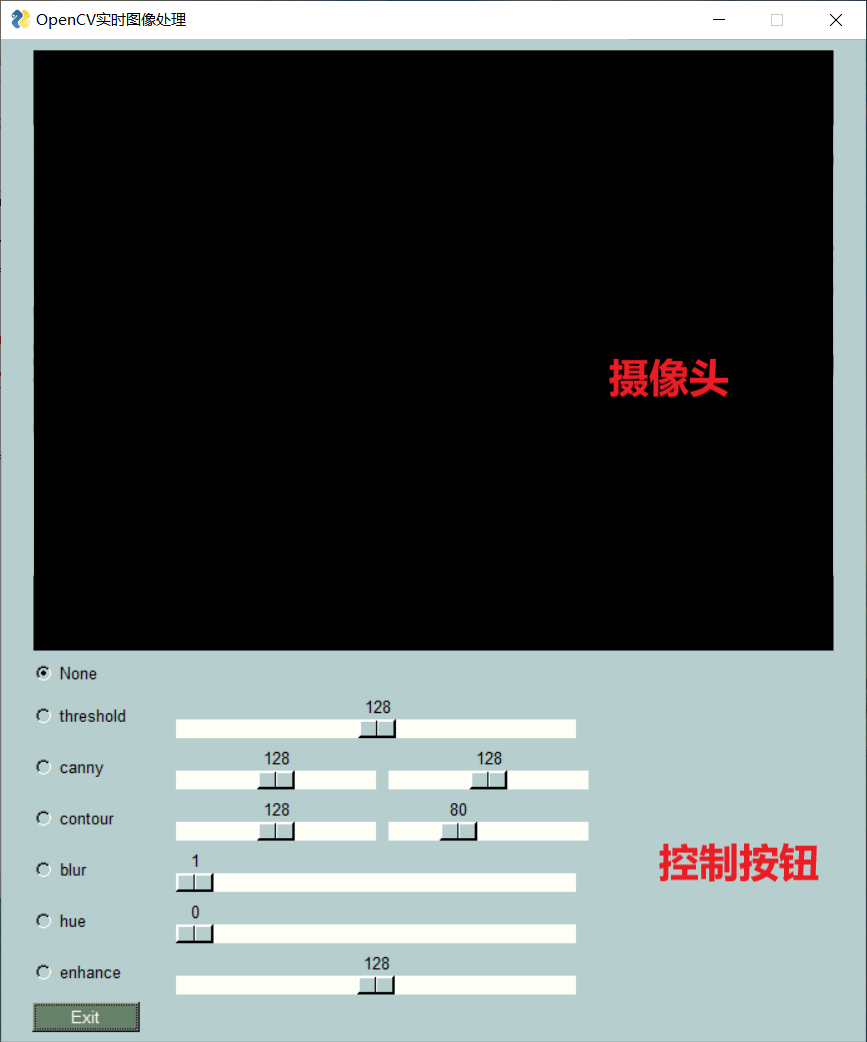GUI代码如下所示：

    #背景色
sg.theme('LightGreen')

#定义窗口布局
layout = [
[sg.Image(filename='', key='image')],
sg.Slider((0, 255), 128, 1, orientation='h', size=(40, 15), key='thresh_slider')],
sg.Slider((0, 255), 128, 1, orientation='h', size=(20, 15), key='canny_slider_a'),
sg.Slider((0, 255), 128, 1, orientation='h', size=(20, 15), key='canny_slider_b')],
sg.Slider((0, 255), 128, 1, orientation='h', size=(20, 15), key='contour_slider'),
sg.Slider((0, 255), 80, 1, orientation='h', size=(20, 15), key='base_slider')],
sg.Slider((1, 11), 1, 1, orientation='h', size=(40, 15), key='blur_slider')],
sg.Slider((0, 225), 0, 1, orientation='h', size=(40, 15), key='hue_slider')],
sg.Slider((1, 255), 128, 1, orientation='h', size=(40, 15), key='enhance_slider')],
[sg.Button('Exit', size=(10, 1))]
]

#窗口设计
window = sg.Window('OpenCV实时图像处理',
layout,
location=(800, 400),
finalize=True)

# 3、调用摄像头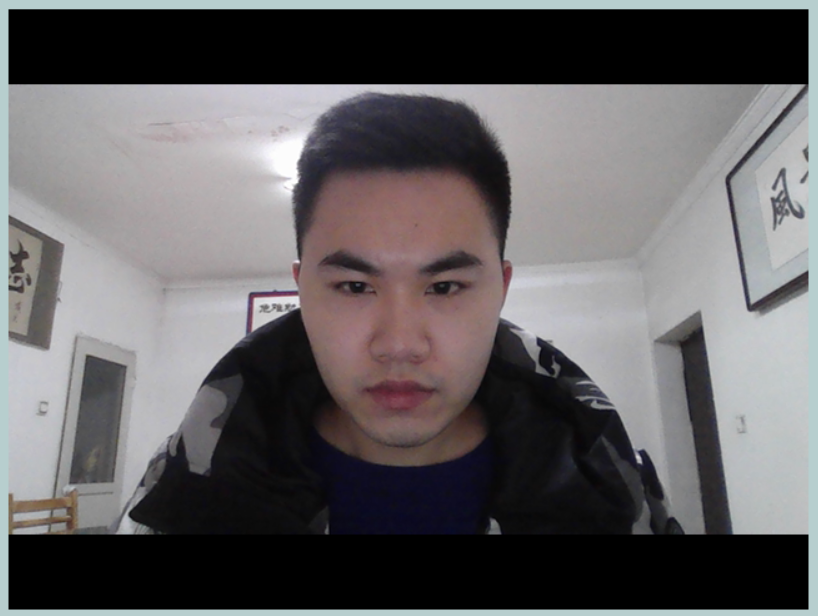#打开内置摄像头
cap = cv2.VideoCapture(0)
while True:

#实时读取图像

#GUI实时更新
imgbytes = cv2.imencode('.png', frame).tobytes()
window['image'].update(data=imgbytes)

window.close()

# 4、实时图像处理

## 4.1、阈值二值化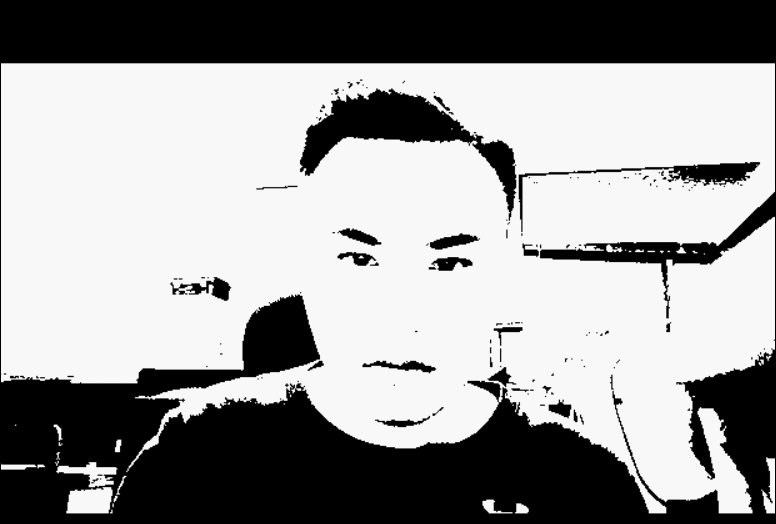代码如下所示：

if values['thresh']:
frame = cv2.cvtColor(frame, cv2.COLOR_BGR2LAB)[:, :, 0]
frame = cv2.threshold(frame, values['thresh_slider'], 255, cv2.THRESH_BINARY)

## 4.2、边缘检测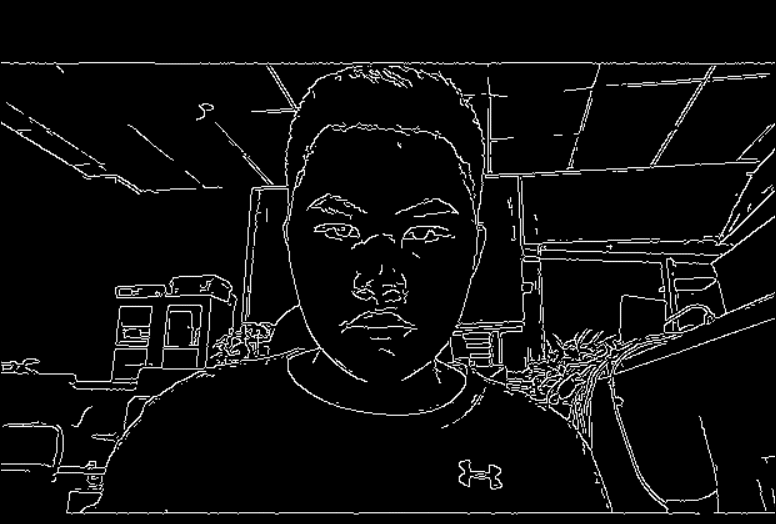if values['canny']:
frame = cv2.Canny(frame, values['canny_slider_a'], values['canny_slider_b'])

## 4.3、轮廓检测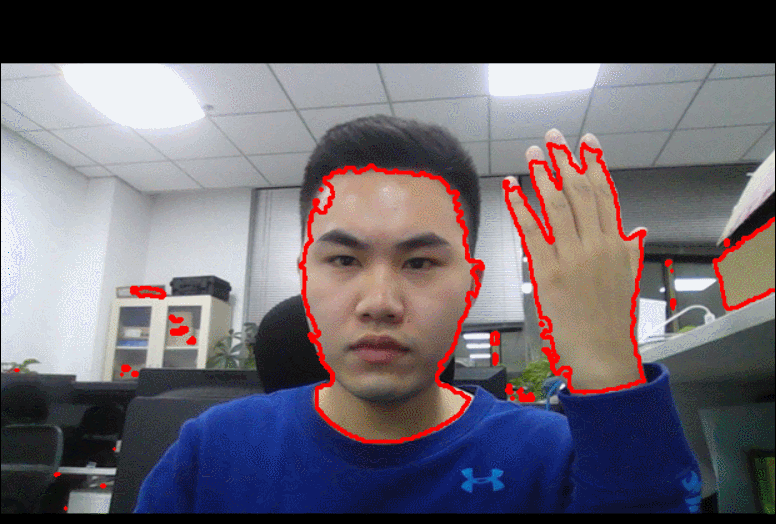代码如下所示：

if values['contour']:
hue = cv2.cvtColor(frame, cv2.COLOR_BGR2HSV)
hue = cv2.GaussianBlur(hue, (21, 21), 1)
hue = cv2.inRange(hue, np.array([values['contour_slider'], values['base_slider'], 40]),
np.array([values['contour_slider'] + 30, 255, 220]))
cnts= cv2.findContours(hue, cv2.RETR_EXTERNAL, cv2.CHAIN_APPROX_SIMPLE)
cv2.drawContours(frame, cnts, -1, (0, 0, 255), 2)


## 4.4、高斯滤波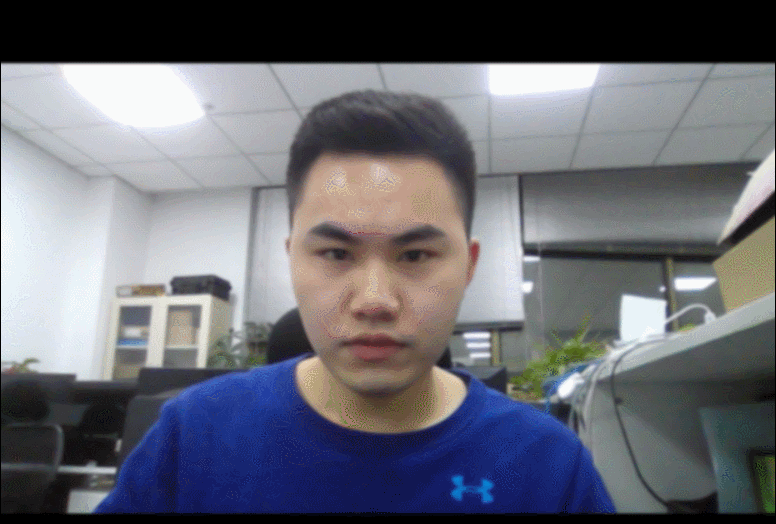代码如下所示：

if values['blur']:
frame = cv2.GaussianBlur(frame, (21, 21), values['blur_slider'])


## 4.5、色彩转换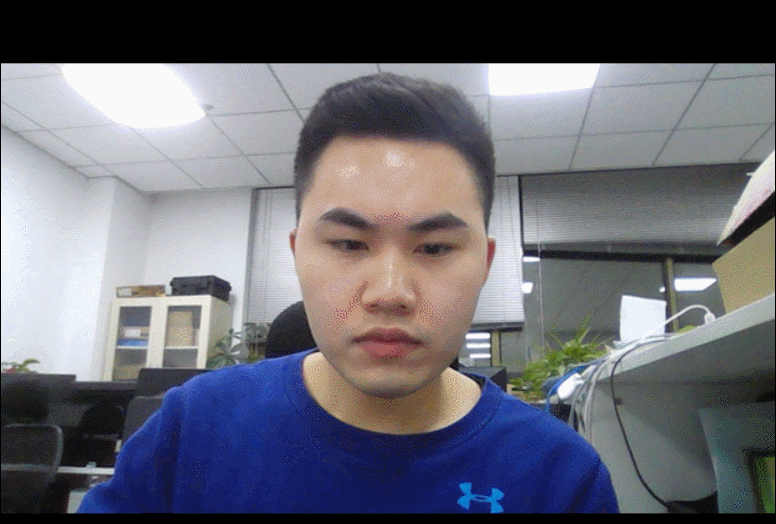代码如下所示：

if values['hue']:
frame = cv2.cvtColor(frame, cv2.COLOR_BGR2HSV)
frame[:, :, 0] += int(values['hue_slider'])
frame = cv2.cvtColor(frame, cv2.COLOR_HSV2BGR)

## 4.6、调节对比度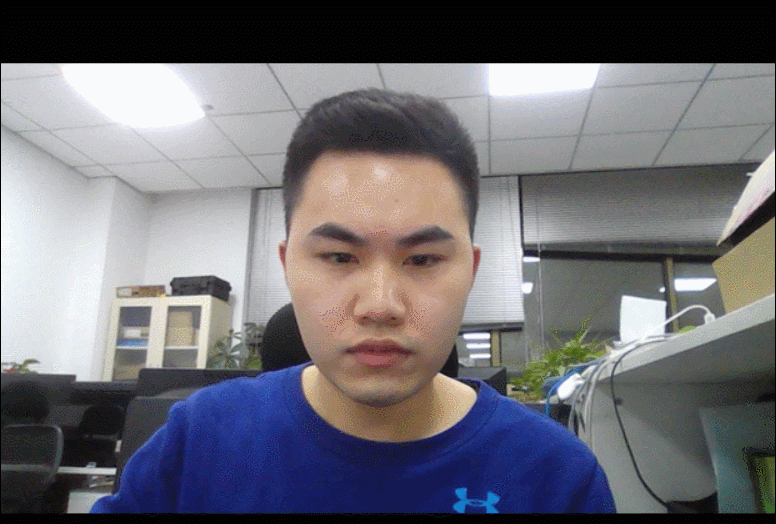代码如下所示：

if values['enhance']:
enh_val = values['enhance_slider'] / 40
clahe = cv2.createCLAHE(clipLimit=enh_val, tileGridSize=(8, 8))
lab = cv2.cvtColor(frame, cv2.COLOR_BGR2LAB)
lab[:, :, 0] = clahe.apply(lab[:, :, 0])
frame = cv2.cvtColor(lab, cv2.COLOR_LAB2BGR)


# 5、退出系统

if event == 'Exit' or event is None:
break

## python进行基本的图像处理

2017-02-10 15:28:58 xiao_lxl 阅读数 10040
• ###### Python+OpenCV3.3图像处理视频教程

Python+OpenCV3.3图像处理视频培训课程：该教程基于Python3.6+OpenCV新版本3.3.0详细讲述Python OpenCV图像处理部分内容，包括opencv人脸识别、人脸检测、数字验证码识别等内容。是Python开发者学习图像知识与应用...

30课时 663分钟 6356人学习 贾志刚
免费试看

# 第 1 章　基本的图像操作和处理

## 1.1　PIL：Python图像处理类库

PIL（Python Imaging Library，图像处理类库）提供了通用的图像处理功能，以及大量有用的基本图像操作，比如图像缩放、裁剪、旋转、颜色转换等。PIL 是免费的，可以从http://www.pythonware.com/products/pil/ 下载。

from PIL import Image

pil_im = Image.open('empire.jpg')


pil_im = Image.open('empire.jpg').convert('L')### 1.1.1　转换图像格式

from PIL import Image
import os

for infile in filelist:
outfile = os.path.splitext(infile) + ".jpg"
if infile != outfile:
try:
Image.open(infile).save(outfile)
except IOError:
print "cannot convert", infile


PIL 的 open() 函数用于创建 PIL 图像对象，save() 方法用于保存图像到具有指定文件名的文件。除了后缀变为“.jpg”，上述代码的新文件名和原文件名相同。PIL 是个足够智能的类库，可以根据文件扩展名来判定图像的格式。PIL 函数会进行简单的检查，如果文件不是 JPEG 格式，会自动将其转换成 JPEG 格式；如果转换失败，它会在控制台输出一条报告失败的消息。

import os
def get_imlist(path):

""" 返回目录中所有JPG 图像的文件名列表"""

return [os.path.join(path,f) for f in os.listdir(path) if f.endswith('.jpg')]


### 1.1.2　创建缩略图

pil_im.thumbnail((128,128))


### 1.1.3　复制和粘贴图像区域

box = (100,100,400,400)
region = pil_im.crop(box)


region = region.transpose(Image.ROTATE_180)
pil_im.paste(region,box)


### 1.1.4　调整尺寸和旋转

out = pil_im.resize((128,128))


out = pil_im.rotate(45)


## 1.2　Matplotlib

### 1.2.1　绘制图像、点和线

from PIL import Image
from pylab import *

# 读取图像到数组中
im = array(Image.open('empire.jpg'))

# 绘制图像
imshow(im)

# 一些点
x = [100,100,400,400]
y = [200,500,200,500]

# 使用红色星状标记绘制点
plot(x,y,'r*')

# 绘制连接前两个点的线
plot(x[:2],y[:2])

# 添加标题，显示绘制的图像
title('Plotting: "empire.jpg"')
show()


axis('off')plot(x,y)         # 默认为蓝色实线
plot(x,y,'r*')    # 红色星状标记
plot(x,y,'go-')   # 带有圆圈标记的绿线
plot(x,y,'ks:')   # 带有正方形标记的黑色点线


'b'

'g'

'r'

'c'

'm'

'y'

'k'

'w'

'-'

'--'

':'

'.'

'o'

's'

'*'

'+'

'x'

### 1.2.2　图像轮廓和直方图

from PIL import Image
from pylab import *

# 读取图像到数组中
im = array(Image.open('empire.jpg').convert('L'))

# 新建一个图像
figure()
# 不使用颜色信息
gray()
# 在原点的左上角显示轮廓图像
contour(im, origin='image')
axis('equal')
axis('off')


figure()
hist(im.flatten(),128)
show()


hist() 函数的第二个参数指定小区间的数目。需要注意的是，因为 hist() 只接受一维数组作为输入，所以我们在绘制图像直方图之前，必须先对图像进行压平处理。flatten() 方法将任意数组按照行优先准则转换成一维数组。图 1-3 为等轮廓线和直方图图像。### 1.2.3　交互式标注

from PIL import Image
from pylab import *

im = array(Image.open('empire.jpg'))
imshow(im)
x = ginput(3)
print 'you clicked:',x
show()


## 1.3　NumPy

NumPyhttp://www.scipy.org/NumPy/）是非常有名的 Python 科学计算工具包，其中包含了大量有用的思想，比如数组对象（用来表示向量、矩阵、图像等）以及线性代数函数。NumPy 中的数组对象几乎贯穿用于本书的所有例子中 1 数组对象可以帮助你实现数组中重要的操作，比如矩阵乘积、转置、解方程系统、向量乘积和归一化，这为图像变形、对变化进行建模、图像分类、图像聚类等提供了基础。

1PyLab 实际上包含 NumPy 的一些内容，如数组类型。这也是我们能够在 1.2 节使用数组类型的原因。

NumPy 可以从 http://www.scipy.org/Download 免费下载，在线说明文档（http://docs.scipy.org/doc/numpy/）包含了你可能遇到的大多数问题的答案。关于 NumPy 的更多内容，请参考开源书籍 。

### 1.3.1　图像数组表示

im = array(Image.open('empire.jpg'))
print im.shape, im.dtype

im = array(Image.open('empire.jpg').convert('L'),'f')
print im.shape, im.dtype


(800, 569, 3) uint8
(800, 569) float32


value = im[i,j,k]


im[i,:] = im[j,:]      # 将第 j 行的数值赋值给第 i 行
im[:,i] = 100          # 将第 i 列的所有数值设为100
im[:100,:50].sum()     # 计算前100 行、前 50 列所有数值的和
im[50:100,50:100]      # 50~100 行，50~100 列（不包括第 100 行和第 100 列）
im[i].mean()           # 第 i 行所有数值的平均值
im[:,-1]               # 最后一列
im[-2,:] (or im[-2])   # 倒数第二行


### 1.3.2　灰度变换

from PIL import Image
from numpy import *

im = array(Image.open('empire.jpg').convert('L'))

im2 = 255 - im # 对图像进行反相处理

im3 = (100.0/255) * im + 100 # 将图像像素值变换到100...200 区间

im4 = 255.0 * (im/255.0)**2 # 对图像像素值求平方后得到的图像


print int(im.min()), int(im.max())2 255
0 253
100 200
0 255


array() 变换的相反操作可以使用 PIL 的 fromarray() 函数完成：

pil_im = Image.fromarray(im)


pil_im = Image.fromarray(uint8(im))


### 1.3.3　图像缩放

NumPy 的数组对象是我们处理图像和数据的主要工具。想要对图像进行缩放处理没有现成简单的方法。我们可以使用之前 PIL 对图像对象转换的操作，写一个简单的用于图像缩放的函数。把下面的函数添加到 imtool.py 文件里：

def imresize(im,sz):
""" 使用PIL 对象重新定义图像数组的大小"""
pil_im = Image.fromarray(uint8(im))

return array(pil_im.resize(sz))


### 1.3.4　直方图均衡化

def histeq(im,nbr_bins=256):
""" 对一幅灰度图像进行直方图均衡化"""

# 计算图像的直方图
imhist,bins = histogram(im.flatten(),nbr_bins,normed=True)
cdf = imhist.cumsum() # 累积分布函数
cdf = 255 * cdf / cdf[-1] # 归一化

# 使用累积分布函数的线性插值，计算新的像素值
im2 = interp(im.flatten(),bins[:-1],cdf)

return im2.reshape(im.shape), cdf


from PIL import Image
from numpy import *

im = array(Image.open('AquaTermi_lowcontrast.jpg').convert('L'))
im2,cdf = imtools.histeq(im)### 1.3.5　图像平均

def compute_average(imlist):
""" 计算图像列表的平均图像"""

# 打开第一幅图像，将其存储在浮点型数组中
averageim = array(Image.open(imlist), 'f')

for imname in imlist[1:]:
try:
averageim += array(Image.open(imname))
except:
print imname + '...skipped'
averageim /= len(imlist)

# 返回uint8 类型的平均图像
return array(averageim, 'uint8')


### 1.3.6　图像的主成分分析（PCA）

PCA（Principal Component Analysis，主成分分析）是一个非常有用的降维技巧。它可以在使用尽可能少维数的前提下，尽量多地保持训练数据的信息，在此意义上是一个最佳技巧。即使是一幅 100×100 像素的小灰度图像，也有 10 000 维，可以看成 10 000 维空间中的一个点。一兆像素的图像具有百万维。由于图像具有很高的维数，在许多计算机视觉应用中，我们经常使用降维操作。PCA 产生的投影矩阵可以被视为将原始坐标变换到现有的坐标系，坐标系中的各个坐标按照重要性递减排列。

from PIL import Image
from numpy import *

def pca(X):
""" 主成分分析：
输入：矩阵X ，其中该矩阵中存储训练数据，每一行为一条训练数据
返回：投影矩阵（按照维度的重要性排序）、方差和均值"""

# 获取维数
num_data,dim = X.shape

# 数据中心化
mean_X = X.mean(axis=0)
X = X - mean_X

if dim>num_data:
# PCA- 使用紧致技巧
M = dot(X,X.T) # 协方差矩阵
e,EV = linalg.eigh(M) # 特征值和特征向量
tmp = dot(X.T,EV).T # 这就是紧致技巧
V = tmp[::-1] # 由于最后的特征向量是我们所需要的，所以需要将其逆转
S = sqrt(e)[::-1] # 由于特征值是按照递增顺序排列的，所以需要将其逆转
for i in range(V.shape):
V[:,i] /= S
else:
# PCA- 使用SVD 方法
U,S,V = linalg.svd(X)
V = V[:num_data] # 仅仅返回前nun_data 维的数据才合理

# 返回投影矩阵、方差和均值
return V,S,mean_X


2免费字体图像库由 Martin Solli 收集并上传（http://webstaff.itn.liu.se/~marso/）。

from PIL import Image
from numpy import *
from pylab import *
import pca

im = array(Image.open(imlist)) # 打开一幅图像，获取其大小
m,n = im.shape[0:2] # 获取图像的大小
imnbr = len(imlist) # 获取图像的数目

# 创建矩阵，保存所有压平后的图像数据
immatrix = array([array(Image.open(im)).flatten()
for im in imlist],'f')

# 执行 PCA 操作
V,S,immean = pca.pca(immatrix)

# 显示一些图像（均值图像和前 7 个模式）
figure()
gray()
subplot(2,4,1)
imshow(immean.reshape(m,n))
for i in range(7):
subplot(2,4,i+2)
imshow(V[i].reshape(m,n))

show()### 1.3.7　使用pickle模块

# 保存均值和主成分数据
f = open('font_pca_modes.pkl', 'wb')
pickle.dump(immean,f)
pickle.dump(V,f)
f.close()


# 载入均值和主成分数据
f = open('font_pca_modes.pkl', 'rb')
f.close()


# 打开文件并保存
with open('font_pca_modes.pkl', 'wb') as f:
pickle.dump(immean,f)
pickle.dump(V,f)


# 打开文件并载入
with open('font_pca_modes.pkl', 'rb') as f:


savetxt('test.txt',x,'%i')


x = loadtxt('test.txt')


## 1.4　SciPy

SciPyhttp://scipy.org/） 是建立在 NumPy 基础上，用于数值运算的开源工具包。SciPy 提供很多高效的操作，可以实现数值积分、优化、统计、信号处理，以及对我们来说最重要的图像处理功能。接下来，本节会介绍 SciPy 中大量有用的模块。SciPy 是个开源工具包，可以从http://scipy.org/Download 下载。

### 1.4.1　图像模糊

Iσ = I*Gσ

SciPy 有用来做滤波操作的 scipy.ndimage.filters 模块。该模块使用快速一维分离的方式来计算卷积。你可以像下面这样来使用它：

from PIL import Image
from numpy import *
from scipy.ndimage import filters

im = array(Image.open('empire.jpg').convert('L'))
im2 = filters.gaussian_filter(im,5)


im = array(Image.open('empire.jpg'))
im2 = zeros(im.shape)
for i in range(3):
im2[:,:,i] = filters.gaussian_filter(im[:,:,i],5)
im2 = uint8(im2)


im2 = array(im2,'uint8')### 1.4.2　图像导数

α=arctan2(IyIx)

Ix=I*Dx 和 Iy=I*Dy

和

和

from PIL import Image
from numpy import *
from scipy.ndimage import filters

im = array(Image.open('empire.jpg').convert('L'))

# Sobel 导数滤波器
imx = zeros(im.shape)
filters.sobel(im,1,imx)

imy = zeros(im.shape)
filters.sobel(im,0,imy)

magnitude = sqrt(imx**2+imy**2)Ix=I*Gσx 和 Iy=I*Gσy

sigma = 5 # 标准差

imx = zeros(im.shape)
filters.gaussian_filter(im, (sigma,sigma), (0,1), imx)

imy = zeros(im.shape)
filters.gaussian_filter(im, (sigma,sigma), (1,0), imy)### 1.4.3　形态学：对象计数

scipy.ndimage 中的 morphology 模块可以实现形态学操作。你可以使用 scipy.ndimage 中的measurements 模块来实现二值图像的计数和度量功能。下面通过一个简单的例子介绍如何使用它们。

3这个图像实际上是图像“分割”后的结果。如果你想知道该图像是如何创建的，可以查看 9.3 节。

from scipy.ndimage import measurements,morphology

# 载入图像，然后使用阈值化操作，以保证处理的图像为二值图像
im = array(Image.open('houses.png').convert('L'))
im = 1*(im<128)

labels, nbr_objects = measurements.label(im)
print "Number of objects:", nbr_objects



# 形态学开操作更好地分离各个对象
im_open = morphology.binary_opening(im,ones((9,5)),iterations=2)

labels_open, nbr_objects_open = measurements.label(im_open)
print "Number of objects:", nbr_objects_open


binary_opening() 函数的第二个参数指定一个数组结构元素。该数组表示以一个像素为中心时，使用哪些相邻像素。在这种情况下，我们在 y 方向上使用 9 个像素（上面 4 个像素、像素本身、下面 4 个像素），在 x 方向上使用 5 个像素。你可以指定任意数组为结构元素，数组中的非零元素决定使用哪些相邻像素。参数 iterations 决定执行该操作的次数。你可以尝试使用不同的迭代次数 iterations 值，看一下对象的数目如何变化。你可以在图 1-12c 与图 1-12d 中查看经过开操作后的图像，以及相应的标签图像。正如你想象的一样，binary_closing() 函数实现相反的操作。我们将该函数和在 morphology 和 measurements 模块中的其他函数的用法留作练习。你可以从 scipy.ndimage 模块文档 http://docs.scipy.org/doc/scipy/reference/ndimage.html 中了解关于这些函数的更多知识。### 1.4.4　一些有用的SciPy模块

SciPy 中包含一些用于输入和输出的实用模块。下面介绍其中两个模块：io 和 misc

1. 读写.mat文件

如果你有一些数据，或者在网上下载到一些有趣的数据集，这些数据以 Matlab 的 .mat 文件格式存储，那么可以使用 scipy.io 模块进行读取。

data = scipy.io.loadmat('test.mat')


上面代码中，data 对象包含一个字典，字典中的键对应于保存在原始 .mat 文件中的变量名。由于这些变量是数组格式的，因此可以很方便地保存到 .mat 文件中。你仅需创建一个字典（其中要包含你想要保存的所有变量），然后使用 savemat() 函数：

data = {}
data['x'] = x
scipy.io.savemat('test.mat',data)


因为上面的脚本保存的是数组 x，所以当读入到 Matlab 中时，变量的名字仍为 x。关于scipy.io 模块的更多内容，请参见在线文档http://docs.scipy.org/doc/scipy/reference/io.html

2. 以图像形式保存数组

因为我们需要对图像进行操作，并且需要使用数组对象来做运算，所以将数组直接保存为图像文件 4 非常有用。本书中的很多图像都是这样的创建的。

imsave() 函数可以从 scipy.misc 模块中载入。要将数组 im 保存到文件中，可以使用下面的命令：

from scipy.misc import imsave
imsave('test.jpg',im)


scipy.misc 模块同样包含了著名的 Lena 测试图像：

lena = scipy.misc.lena()


该脚本返回一个 512×512 的灰度图像数组。

4所有 Pylab 图均可保存为多种图像格式，方法是点击图像窗口中的“保存”按钮。

## 1.5　高级示例：图像去噪

ROF 模型的数学基础和处理技巧非常高深，不在本书讲述范围之内。在讲述如何基于 Chambolle 提出的算法  实现 ROF 求解器之前，本书首先简要介绍一下 ROF 模型。

（1.1）

from numpy import *

def denoise(im,U_init,tolerance=0.1,tau=0.125,tv_weight=100):
""" 使用A. Chambolle（2005）在公式（11）中的计算步骤实现Rudin-Osher-Fatemi（ROF）去噪模型

输入：含有噪声的输入图像（灰度图像）、U 的初始值、TV 正则项权值、步长、停业条件

输出：去噪和去除纹理后的图像、纹理残留"""

m,n = im.shape # 噪声图像的大小

# 初始化
U = U_init
Px = im # 对偶域的x 分量
Py = im # 对偶域的y 分量
error = 1

while (error > tolerance):
Uold = U

# 原始变量的梯度
GradUx = roll(U,-1,axis=1)-U # 变量U 梯度的x 分量
GradUy = roll(U,-1,axis=0)-U # 变量U 梯度的y 分量

# 更新对偶变量
NormNew = maximum(1,sqrt(PxNew**2+PyNew**2))

Px = PxNew/NormNew # 更新x 分量（对偶）
Py = PyNew/NormNew # 更新y 分量（对偶）

# 更新原始变量
RxPx = roll(Px,1,axis=1) # 对x 分量进行向右x 轴平移
RyPy = roll(Py,1,axis=0) # 对y 分量进行向右y 轴平移

DivP = (Px-RxPx)+(Py-RyPy) # 对偶域的散度
U = im + tv_weight*DivP # 更新原始变量

# 更新误差
error = linalg.norm(U-Uold)/sqrt(n*m);

return U,im-U # 去噪后的图像和纹理残余


from numpy import *
from numpy import random
from scipy.ndimage import filters
import rof

# 使用噪声创建合成图像
im = zeros((500,500))
im[100:400,100:400] = 128
im[200:300,200:300] = 255
im = im + 30*random.standard_normal((500,500))

U,T = rof.denoise(im,im)
G = filters.gaussian_filter(im,10)

# 保存生成结果
from scipy.misc import imsave
imsave('synth_rof.pdf',U)
imsave('synth_gaussian.pdf',G)from PIL import Image
from pylab import *
import rof

im = array(Image.open('empire.jpg').convert('L'))
U,T = rof.denoise(im,im)

figure()
gray()
imshow(U)
axis('equal')
axis('off')
show()## 练习

1. 如图 1-9 所示，将一幅图像进行高斯模糊处理。随着 σ 的增加，绘制出图像轮廓。在你绘制出的图中，图像的轮廓有何变化？你能解释为什么会发生这些变化吗？

3. 除了直方图均衡化，商图像是另一种图像归一化的方法。商图像可以通过除以模糊后的图像I/(IGσ) 获得。尝试使用该方法，并使用一些样本图像进行验证。

4. 使用图像梯度，编写一个在图像中获得简单物体（例如，白色背景中的正方形）轮廓的函数。

5. 使用梯度方向和大小检测图像中的线段。估计线段的长度以及线段的参数，并在原始图像中重新绘制该线段。

6. 使用 label() 函数处理二值化图像，并使用直方图和标签图像绘制图像中物体的大小分布。

7. 使用形态学操作处理阈值化图像。在发现一些参数能够产生好的结果后，使用 morphology 模块里面的 center_of_mass() 函数寻找每个物体的中心坐标，将其在图像中绘制出来。

## 代码示例约定

from PIL import Image
from numpy import *
from pylab import *


import numpy as np
import matplotlib.pyplot as plt